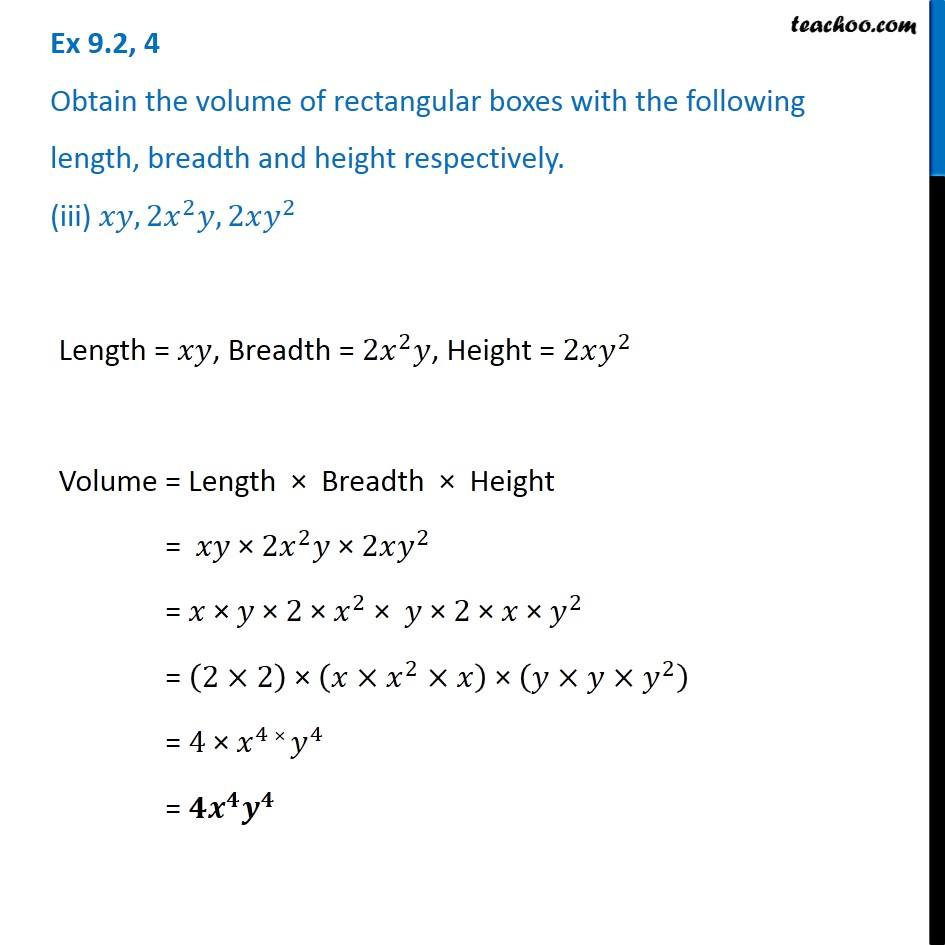Ex 9.2

Chapter 9 Class 8 Algebraic Expressions and Identities
Serial order wiseGet live Maths 1-on-1 Classs - Class 6 to 12

### Transcript

Ex 9.2, 4 Obtain the volume of rectangular boxes with the following length, breadth and height respectively. (iii) 𝑥𝑦, 2𝑥^2 𝑦, 2𝑥𝑦^2 Length = 𝑥𝑦, Breadth = 2𝑥^2 𝑦, Height = 2𝑥𝑦^2 Volume = Length × Breadth × Height = 𝑥𝑦 × 2𝑥^2 𝑦 × 2𝑥𝑦^2 = 𝑥 × 𝑦 × 2 × 𝑥^2 × 𝑦 × 2 × 𝑥 × 𝑦^2 = (2×2) × (𝑥×𝑥^2×𝑥) × (𝑦×𝑦×𝑦^2 ) = 4 × 𝑥^4 × 𝑦^4 = 𝟒𝒙^𝟒 𝒚^𝟒